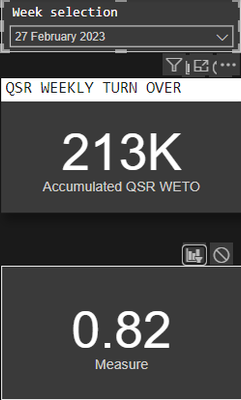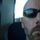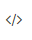cancel
Showing results for
Did you mean:Regular Visitor

## Slicer not filtering Measure

Hello,

I am trying to use a slicer as a pge filter however I have a series of calculated measures that are not filtering. The slicer is working fine for fields on the same table but my measures are pulling information from several other tables. I might be asking a sily question but how can I make this work?2 ACCEPTED SOLUTIONSSuper User

We would need to see your model and how the tables are related to each other, and the DAX in the measures that are unaffected. Post actual text for the DAX, and format it please using DAXFormatter.com - I cannot read that tiny image and cannot debug unformatted DAX like that.

Use this button in the forum post menu to post DAX code:Did my answers help arrive at a solution? Give it a kudos by clicking the Thumbs Up!

DAX is for Analysis. Power Query is for Data Modeling

Proud to be a Super User!

MCSA: BI ReportingSuper User

If the Week Date in the slicer is coming from the OPS Table, it won't work. That table is on the many side of relationships and other tables filter it, but it filters nothing. You could turn on bi-directional filtering, but that is an extraordinarly bad idea. You need a true date table that filters all of your fact tables, then you add the Week date from the date table, and your model should be set up as a Star Schema.

If you want an overview of a date table, see this blog. Create A Dynamic Date Table In Power Query — ehansalytics You can skip the part about making it dynamic. But without a doubt, you need a date table.

You should also get rid of all of those 1:1 relationships and merge those tables in Power Query. A 1:1 relationship is 99.9% of the time 1 table split in two.

Microsoft recommends minimizing use of both Many-to-Many and Bi-Directional Relationships. In other words, unless you are a DAX expert, find another way to remodel your data to conform to a Star Schema and don't use these two features. I avoid them both at all costs.
Microsoft Guidance on Many-To-Many Relationships
Microsoft Guidance on Bi-Directional Relationships
Microsoft Guidance on Importance of Star Schema

Did my answers help arrive at a solution? Give it a kudos by clicking the Thumbs Up!

DAX is for Analysis. Power Query is for Data Modeling

Proud to be a Super User!

MCSA: BI Reporting
6 REPLIES 6Regular Visitor

Hi and sorry about that. Here the Codes:

``Measure = (sum('ROLMON Table'[Food Sales Net])+sum('TB9S Table'[Food Sales Net])+sum('TBAC Table'[Food Sales Net])+sum('TBDE Table'[Food Sales Net])+sum('TBFH Table'[Food Sales Net])+sum('TBMON Table'[Food Sales Net])+sum('TBSC Table'[Food Sales Net])+sum('TLAC Table'[Food Sales Net])+sum('TLMON Table'[Food Sales Net]))/(SUM('ROLMON Table'[Beverage High Net])+SUM('ROLMON Table'[Beverage Low Net])+SUM('TB9S Table'[Beverage High Net])+SUM('TBAC Table'[Beverage High Net])+sum('TBAC Table'[Beverage Low Net])+SUM('TB9S Table'[Beverage Low Net])+sum('TBDE Table'[Beverage High Net])+sum('TBDE Table'[Beverage Low Net])+SUM('TBSC Table'[Beverage High Net])+sum('TBSC Table'[Beverage Low Net])+sum('TLMON Table'[Beverage Low Net])+sum('ROLMON Table'[Food Sales Net])+sum('TB9S Table'[Food Sales Net])+sum('TBAC Table'[Food Sales Net])+sum('TBDE Table'[Food Sales Net])+sum('TBFH Table'[Food Sales Net])+sum('TBMON Table'[Food Sales Net])+sum('TBSC Table'[Food Sales Net])+sum('TLAC Table'[Food Sales Net])+sum('TLMON Table'[Food Sales Net]))``Super User

Ok, I'll try to look at later. But if anyone wants to look at it here is the readable code formatted by DAXFormatter.

``````Test Measure =
(
SUM ( 'ROLMON Table'[Food Sales Net] )
+ SUM ( 'TB9S Table'[Food Sales Net] )
+ SUM ( 'TBAC Table'[Food Sales Net] )
+ SUM ( 'TBDE Table'[Food Sales Net] )
+ SUM ( 'TBFH Table'[Food Sales Net] )
+ SUM ( 'TBMON Table'[Food Sales Net] )
+ SUM ( 'TBSC Table'[Food Sales Net] )
+ SUM ( 'TLAC Table'[Food Sales Net] )
+ SUM ( 'TLMON Table'[Food Sales Net] )
)
/ (
SUM ( 'ROLMON Table'[Beverage High Net] )
+ SUM ( 'ROLMON Table'[Beverage Low Net] )
+ SUM ( 'TB9S Table'[Beverage High Net] )
+ SUM ( 'TBAC Table'[Beverage High Net] )
+ SUM ( 'TBAC Table'[Beverage Low Net] )
+ SUM ( 'TB9S Table'[Beverage Low Net] )
+ SUM ( 'TBDE Table'[Beverage High Net] )
+ SUM ( 'TBDE Table'[Beverage Low Net] )
+ SUM ( 'TBSC Table'[Beverage High Net] )
+ SUM ( 'TBSC Table'[Beverage Low Net] )
+ SUM ( 'TLMON Table'[Beverage Low Net] )
+ SUM ( 'ROLMON Table'[Food Sales Net] )
+ SUM ( 'TB9S Table'[Food Sales Net] )
+ SUM ( 'TBAC Table'[Food Sales Net] )
+ SUM ( 'TBDE Table'[Food Sales Net] )
+ SUM ( 'TBFH Table'[Food Sales Net] )
+ SUM ( 'TBMON Table'[Food Sales Net] )
+ SUM ( 'TBSC Table'[Food Sales Net] )
+ SUM ( 'TLAC Table'[Food Sales Net] )
+ SUM ( 'TLMON Table'[Food Sales Net] )
)
``````

Did my answers help arrive at a solution? Give it a kudos by clicking the Thumbs Up!

DAX is for Analysis. Power Query is for Data Modeling

Proud to be a Super User!

MCSA: BI ReportingRegular Visitor

I womder if it has to do the fact that the Week Date is a sigle date for a full week and the data from the tables added are daily values.Super User

If the Week Date in the slicer is coming from the OPS Table, it won't work. That table is on the many side of relationships and other tables filter it, but it filters nothing. You could turn on bi-directional filtering, but that is an extraordinarly bad idea. You need a true date table that filters all of your fact tables, then you add the Week date from the date table, and your model should be set up as a Star Schema.

If you want an overview of a date table, see this blog. Create A Dynamic Date Table In Power Query — ehansalytics You can skip the part about making it dynamic. But without a doubt, you need a date table.

You should also get rid of all of those 1:1 relationships and merge those tables in Power Query. A 1:1 relationship is 99.9% of the time 1 table split in two.

Microsoft recommends minimizing use of both Many-to-Many and Bi-Directional Relationships. In other words, unless you are a DAX expert, find another way to remodel your data to conform to a Star Schema and don't use these two features. I avoid them both at all costs.
Microsoft Guidance on Many-To-Many Relationships
Microsoft Guidance on Bi-Directional Relationships
Microsoft Guidance on Importance of Star Schema

Did my answers help arrive at a solution? Give it a kudos by clicking the Thumbs Up!

DAX is for Analysis. Power Query is for Data Modeling

Proud to be a Super User!

MCSA: BI ReportingRegular Visitor

AMazing I'll get cracking on it many thanks!!Super User

We would need to see your model and how the tables are related to each other, and the DAX in the measures that are unaffected. Post actual text for the DAX, and format it please using DAXFormatter.com - I cannot read that tiny image and cannot debug unformatted DAX like that.

Use this button in the forum post menu to post DAX code:Did my answers help arrive at a solution? Give it a kudos by clicking the Thumbs Up!

DAX is for Analysis. Power Query is for Data Modeling

Proud to be a Super User!

MCSA: BI Reporting